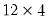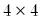Command Reference : Matrix Language Reference

 @kronecker
Kronecker product.
Syntax: @kronecker(m1, m2)
m1: matrix, vector, sym
m2: matrix, vector, sym
Return: matrix
Calculates the Kronecker product of the two matrix objects, m1 and m2.
The resulting matrix has a number of rows equal to the product of the numbers of rows of the two matrix objects and a number of columns equal to the product of the numbers of columns of the two matrix objects.
The elements of the resulting matrix consist of submatrices consisting of an element of the first matrix object multiplied by the entire second matrix object.
Examples
matrix m3 = @kronecker(m1, m2)
produces the Kronecker product of the matrices M1 and M2.
matrix k4 = @kronecker(@fill(1, 2, 3), @ones(4, 4))
generates thematrix formed by taking the Kronecker product of the 3 element vector and thematrix of ones. The matrix consists of the rectangle with the column vector containing (1, 2, 3) repeated 4 times vertically and horizontally.
Cross-references